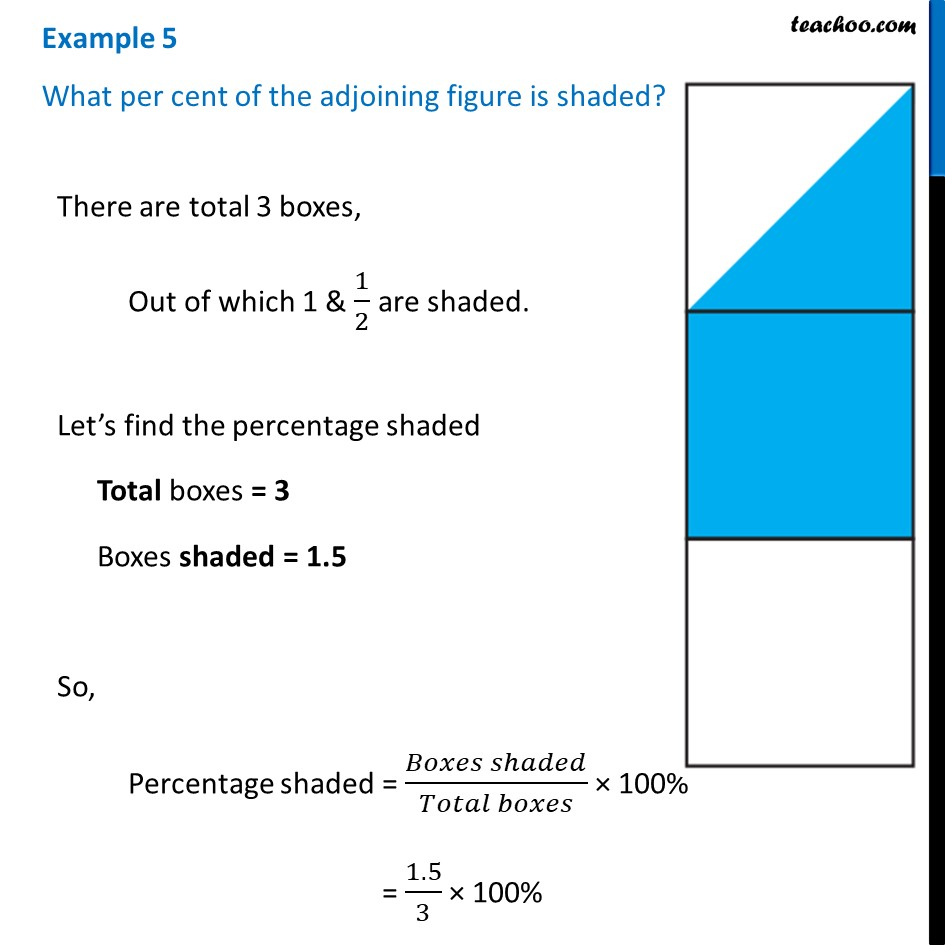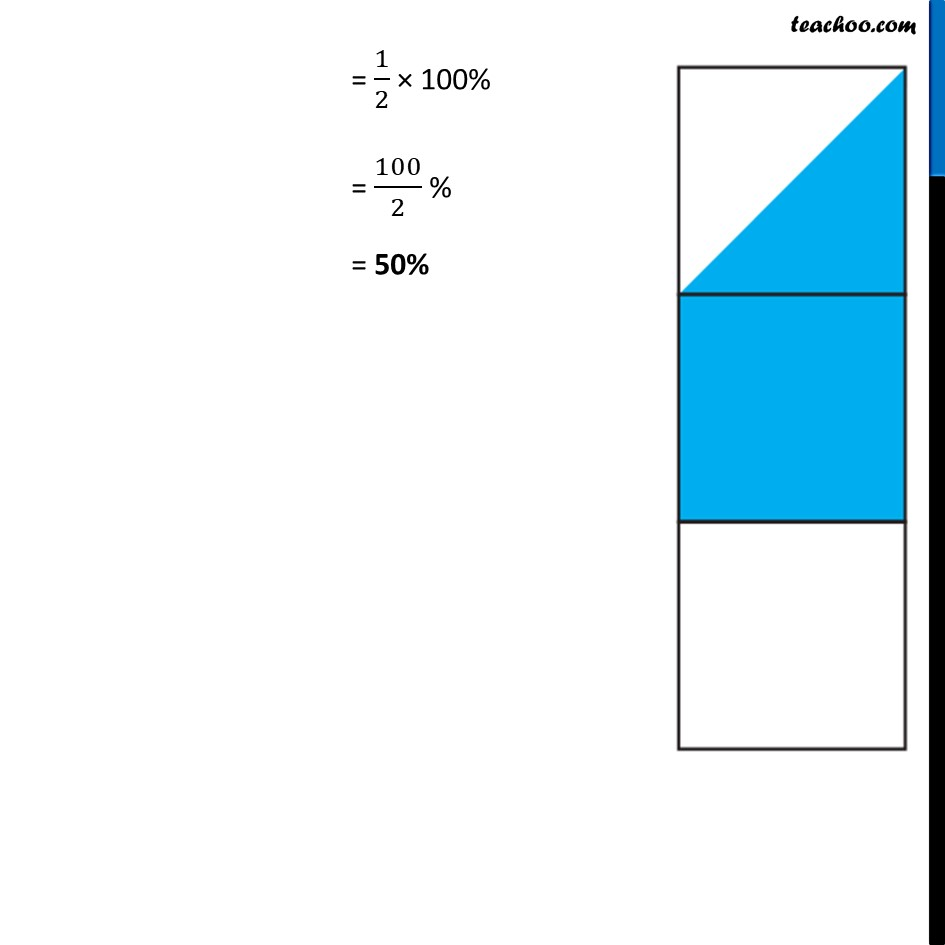Examples

Chapter 7 Class 7 Comparing Quantities
Serial order wiseLearn in your speed, with individual attention - Teachoo Maths 1-on-1 Class

### Transcript

Example 5 What per cent of the adjoining figure is shaded? There are total 3 boxes, Out of which 1 & 1/2 are shaded. Let’s find the percentage shaded Total boxes = 3 Boxes shaded = 1.5 So, Percentage shaded = (𝐵𝑜𝑥𝑒𝑠 𝑠ℎ𝑎𝑑𝑒𝑑)/(𝑇𝑜𝑡𝑎𝑙 𝑏𝑜𝑥𝑒𝑠) × 100% = 1.5/3 × 100% = 1/2 × 100% = 100/2 % = 50%